# Tools extension - my second extension [FREE]

### Write a short description

Tools Extension - build specially for converting measurements of several things. You will also receive a list of measurements, and their values.
This extension contains function to generate random number from 0 to the number that user choose and generating radom numbers and strings.

### Add a picture of all the blocks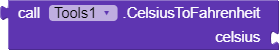==Convert Celsius to Fahrenheit==.
Input: ~double or integer number
Returns: ~double number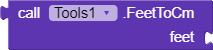==Convert Feet to Cm.==
Input: ~double or integer number
Returns: ~double number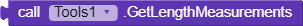==Get all the length measurements as list.
Index 1 - Mile, index 2 - Feet, index 3 - Yard, index 4 - Inch. (This returns how much in one Km (index 1) or Cm (indexes 2, 3 and 4).)==
Returns: ~list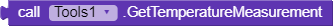==Get all the temperature measurements. Because it is only one (fahrenheit), it’s returned as double number.==
Returns: ~double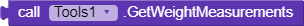==Get all the weight measurements as list.
Index 1 - Lb, index 2 - Oz. (This returns how much in one Kg.)==
Returns: ~list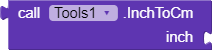==Convert Inch to Cm.==
Input: ~double or integer number
Returns: ~double number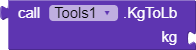==Convert Kg to Lb.==
Input: ~double or integer number
Returns: ~double number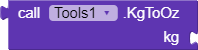==Convert Kg to Oz.==
Input: ~double or integer number
Returns: ~double number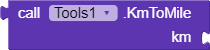==Convert Km to Mile.==
Input: ~double or integer number
Returns: ~double number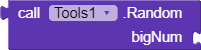==Generate random number from 0 to bigNum (0 included and bigNum-1 included).
For 54, the numbers will 0 to 53.==
Input: ~integer number
Returns: ~integer number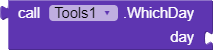==Enter number between 1 to 7 (number of day) and this function will return the name of the enterd day.==
Input: ~integer number
Returns: ~string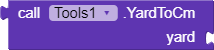==Convert Yard to Cm.==
Input: ~double or integer number
Returns: ~double number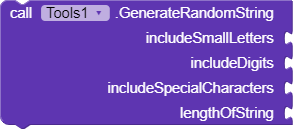==Generate random string from A-Z. Use it for serial number or something else.
Mark the includeSmallLetters to true if you want them.
Mark the includeDigits to true if you want them.
length is number, from 1 to your choice. It can’t be empty! by default the length is 16.==

Inputs:
includeSmallLetters: ~boolean
includeDigits: ~boolean
includeSpecialCharacters: ~boolean
lengthOfString: ~integer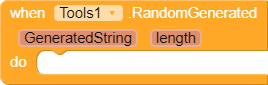==Raises when the generade operation done. The random string will be here in varible.==
==The varibale returns String, and the lenght returns Integer.==

com.matanel.Tools.aix (11.9 ק״ב)

Relased: 2020-10-01T21:15:00Z
Updated: 2022-12-20T11:15:00Z

Credit goes to who helping me a lot, all the time - @Mohamed_Tamer.
Thanks a lot.
Hope you enjoy.
your best, and happyoding!

17 Likes

Very useful extensionThanks7 Likes

Excellent work @Matanel325.
It is a good extension which can be used to convert various units.

Keep koding…
All the best.

2 Likes

Awesome3 Likes

Wow! That’s an interesting extension for some type of apps!! Great job!2 Likes

Update: version 2 relased. (free version).
the paid version will be soon when I will find a payment solution.

2 Likes

Update: I put paypal link for payment
the price is 6 USD (20 ILS).

1 Like

Question: which tools do you want to see in the next release?
I want to make more blocks but for now I don’t have some ideasThank you.

Nice start! But which of the provided functions aren’t currently possible with a little piece of math code in kodular?
Also the random things can be easily done with a list containing all letters and symbols of your keyboard and a math random block.

2 Likes

@Django_s_Android_App
Yea I know that, I did this for fun and to learn a little the basics of Java (I don’t really know and this is how I’m learning).
Do you have any suggestions to next methods\extensions that I can practice making them?
Thanks.

I updated my post, take a look